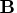Previous Page | Next Page

 Language Reference

## Transpose Operator:   `

transposes a matrix

matrix`

The transpose operator (denoted by the backquote ` character) exchanges the rows and columns of matrix, producing the transpose of matrix. For example, if an element in matrix is in the first row and second column, it is in the second row and first column of the transpose; an element in the first row and third column of matrix is in the third row and first column of the transpose, and so on. If matrix contains three rows and two columns, its transpose has two rows and three columns.

For example, the following statements produce the matrix, as shown:

```
a={1 2,
3 4,
5 6};
b=a`;
```

```
B             2 rows      3 cols    (numeric)

1         3         5
2         4         6
```
If your keyboard does not have a backquote character, you can transpose a matrix with the T (transpose) function, documented later in this chapter.Previous Page | Next Page | Top of Page